# Optical Wheel Encoder with DC motor on Arduino

#### grgDek

Joined Mar 20, 2020
15
Hello everyone !
I am new here, glad to found this place !

It's been 4 days i am trying to use an optical wheel encoder (incremential i think), with a DCmotor, both took apart from my useless Canon Pixma Printer. My encoder has a red Led and photoresistor. What i am trying to do is to read the encoder datas in order to drive the motor like a stepper one, knowing its speed, position and direction.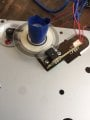I found out the pinout of my encoder by testing its pins with a multimeter in diode mode like explains here : https://reprap.org/wiki/Optical_encoders_01

My encoder pinouts is: A=Led - , B=Led+, C=gnd , D=output 1 , E=+5V , F=output 2. Where the Output 1 is attached to Led- pin (same wire), and Led+ attached to +5V pin (same wire too), so i have 4 wires; 5V(+), Gnd, Output1, Output2.

I am using an arduino Uno with a L293D driver for the motor.

I have tested a dozen different wiring and codes from several forums, but each time the encoder return nothing or zero. Below, some wiring&code that i've tried out.

1/ DC motor + Driver L293D + OpticalEncoder + arduinoUno + 9V powerSupply(for the motor)

wiring (https://andrewjkramer.net/motor-encoders-arduino/):Mine is a bit different cause i am not using the same driver, and my encoder has 4 pins.

code:
C++:
// Motor connections

int enB = 5;

int in3 = 6;

int in4 = 4;

// Encoder Output connections

// from https://scidle.com/how-to-use-mouse-scroll-wheel-encoder-with-arduino/

byte AInput = 2;

byte BInput = 3;

byte lastState = 0;

byte steps = 0;

int  cw = 0;

byte AState = 0;

byte BState = 0;

byte State = 0;

void setup() {

Serial.begin(9600);

// Set all the motor control pins to outputs

pinMode(enB, OUTPUT);

pinMode(in3, OUTPUT);

pinMode(in4, OUTPUT);

// Turn off motors - Initial state

digitalWrite(in3, LOW);

digitalWrite(in4, LOW);

// set the Encoders pins to Input

pinMode(AInput, INPUT_PULLUP); //tried with 'INPUT'

pinMode(BInput, INPUT_PULLUP); // tried with 'INPUT'

}

void loop() {

//directionControl();

//delay(1000);

//speedControl();

//delay(1000);

delay (2000);

}

// this function is for encoder

State = AState | BState;

if (lastState != State){

switch (State) {

case 0:

if (lastState == 2){

steps++;

cw = 1;

}

else if(lastState == 1){

steps--;

cw = -1;

}

break;

case 1:

if (lastState == 0){

steps++;

cw = 1;

}

else if(lastState == 3){

steps--;

cw = -1;

}

break;

case 2:

if (lastState == 3){

steps++;

cw = 1;

}

else if(lastState == 0){

steps--;

cw = -1;

}

break;

case 3:

if (lastState == 1){

steps++;

cw = 1;

}

else if(lastState == 2){

steps--;

cw = -1;

}

break;

}

}

lastState = State;

Serial.print(State);

Serial.print("\t");

Serial.print(cw);

Serial.print("\t");

Serial.println(steps);

delay(1);

}

// This function lets you control spinning direction of motors

void directionControl() {

// Set motors to maximum speed

// For PWM maximum possible values are 0 to 255

analogWrite(enB, 255);

// Turn on motor A & B

digitalWrite(in3, HIGH);

digitalWrite(in4, LOW);

delay(2000);

// Now change motor directions

digitalWrite(in3, LOW);

digitalWrite(in4, HIGH);

delay(2000);

// Turn off motors

digitalWrite(in3, LOW);

digitalWrite(in4, LOW);

}

// This function lets you control speed of the motors

void speedControl() {

// Turn on motors

digitalWrite(in3, LOW);

digitalWrite(in4, HIGH);

// Accelerate from zero to maximum speed

for (int i = 0; i < 256; i++) {

analogWrite(enB, i);

delay(20);

}

// Decelerate from maximum speed to zero

for (int i = 255; i >= 0; --i) {

analogWrite(enB, i);

delay(20);

}

// Now turn off motors

digitalWrite(in3, LOW);

digitalWrite(in4, LOW);

}
Results: The motor behaviour is good according to the code, but the encoder returns only 0 0 0... I tried it without the DCMotor, turning the wheel by hand, but the results are the same...

2/ Simple code and wiring to test only the encoder. OpticalEncoder + arduinoUno +wires.

Wiring:

A(+5V) pin ─> 5V on board

B(Gnd) pin ─> Gnd on board

C(Output1) pin ─> D2 on board (i read somwhere that is interrupt pins)

D(Output2) pin ─> D3 on board (i also tried C pin on D3 and D pin on D2)

Code: (found here: https://forum.arduino.cc/index.php?topic=537859.0)
C++:
define encoderPinA 2

define encoderPinB 3

volatile int counter = 0;

volatile boolean flag;

void setup() {

pinMode(encoderPinA, INPUT);
pinMode(encoderPinB, INPUT);
Serial.begin (9600);
attachInterrupt(encoderPinA, isr_2, CHANGE);
}

void loop() {

if(flag == true){
Serial.println(counter);
flag = false;
}

}
//Interrupts
void isr(){
flag = true;

if(channel_A == HIGH && channel_B == HIGH){
counter++;
// Serial.println("BOTH HIGH = " + counter);
}
else{
counter--;
// Serial.println("ELSE counter = " + counter);
}
}

void isr_2(){
flag = true;
counter = counter -1; //COUNTER CLOCK WISE
}
else{
counter = counter +1; //CW
}
}
else{
counter = counter + 1; //CW
}
else{
counter = counter - 1 ; //CCW
}
}
}

Result: Sometimes 0 0 0, sometimes nothing in the serial monitor

3/ with the same components and same wiring

code:
C++:
//these pins can not be changed 2/3 are special pins
int encoderPin1 = 2;
int encoderPin2 = 3;

volatile int lastEncoded = 0;
volatile long encoderValue = 0;

long lastencoderValue = 0;

int lastMSB = 0;
int lastLSB = 0;

void setup() {
Serial.begin (9600);

pinMode(encoderPin1, INPUT);
pinMode(encoderPin2, INPUT);

digitalWrite(encoderPin1, HIGH); //turn pullup resistor on
digitalWrite(encoderPin2, HIGH); //turn pullup resistor on

//call updateEncoder() when any high/low changed seen
//on interrupt 0 (pin 2), or interrupt 1 (pin 3)
attachInterrupt(0, updateEncoder, CHANGE);
attachInterrupt(1, updateEncoder, CHANGE);

}

void loop(){
//Do stuff here

Serial.println(encoderValue);
delay(1000); //just here to slow down the output, and show it will work  even during a delay
}

void updateEncoder(){
int MSB = digitalRead(encoderPin1); //MSB = most significant bit
int LSB = digitalRead(encoderPin2); //LSB = least significant bit

int encoded = (MSB << 1) |LSB; //converting the 2 pin value to single number
int sum  = (lastEncoded << 2) | encoded; //adding it to the previous encoded value

if(sum == 0b1101 || sum == 0b0100 || sum == 0b0010 || sum == 0b1011) encoderValue ++;
if(sum == 0b1110 || sum == 0b0111 || sum == 0b0001 || sum == 0b1000) encoderValue --;

lastEncoded = encoded; //store this value for next time
}
Results: only 0 0 0

more photos: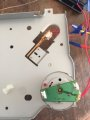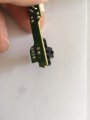I hope some of you could help me figure out what's my mistake(s)Should i make hardware interrupts to set the home of my encoder, how to do so ?
Thanks for reading all this guys !

;:—)}

Joined Jul 18, 2013
22,011
Have you confirmed with a volt meter that the two signal pins are transitioning, IOW for e.g. , do you need a pull-up resistor etc?
I have only used a photo sensor with picmicro, not Arduino.
Max.

#### MrChips

Joined Oct 2, 2009
22,521
Welcome to AAC!

Do you have access to an oscilloscope in order to test for proper signals?
Do you know what is a quadrature encoder?

Joined Jul 18, 2013
22,011
Even with that resolution, you should be able to test with an ordinary voltmeter, if turning VERY slowly.
The OP mentions 'Incremental' so presumably he may know the signal (quadrature)..
Max.

#### grgDek

Joined Mar 20, 2020
15
Have you confirmed with a volt meter that the two signal pins are transitioning, IOW for e.g. , do you need a pull-up resistor etc?
I have only used a photo sensor with picmicro, not Arduino.
Max.
Sorry but I'm not familiar enough with electronic vocabulary, what is IOW ? I read that Pull-up resistor are commonly used between micro-controller and the gnd, so i my case, should i had a resitor between 5V+ and the pin on the encoder ?

It is probably a quadrature encoder. This describes using a quad encoder with arduino, though the encoder is a manual one but the effect is the same.
Thanks for your answer ! I 've already tried with this link, It is even on of the first i've used :—) But unfortunatly i wasn't successful with this one yet...

Welcome to AAC!

Do you have access to an oscilloscope in order to test for proper signals?
Do you know what is a quadrature encoder?
Thanks for your answer ! I don't have access to oscilloscope. Yes i read a lot of documentation about it, I understood that it's about the signal shape and that the encoder compares both signals from outputs to know if the motor is turning CW or CCW, but i didn't dig any deeper.

Even with that resolution, you should be able to test with an ordinary voltmeter, if turning VERY slowly.
The OP mentions 'Incremental' so presumably he may know the signal (quadrature)..
Max.
Yes I will try this ! Sounds good idea, should I do that in diode mode or any other mode ? What information should I read ?
Yep I learned about different kind of encoder and signals :—)

Thanks for your answers, I will try out that tommorow and I will let you know :—)

#### AlbertHall

Joined Jun 4, 2014
10,778
Thanks for your answers, I will try out that tommorow and I will let you know :—)
With a voltmeter.

#### MrChips

Joined Oct 2, 2009
22,521
Ok. Glad to know where you stand.

You can build a simple logic state monitor using a transistor or a CMOS logic gate.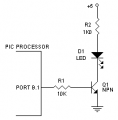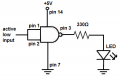Just about any logic gate will do. The only difference is that the LED may be on for the opposite logic state.

With a logic tester such as this (you will need two logic testers) you can examine the logic outputs A and B of the quadrature encoder as you slowly rotate the disc.

Joined Jul 18, 2013
22,011
Sorry but I'm not familiar enough with electronic vocabulary, what is IOW ? I read that Pull-up resistor are commonly used between micro-controller and the gnd, so i my case, should i had a resitor between 5V+ and the pin on the encoder ?
IOW -In Other Words'
I don't know what the input consists of in Arduino, but generally a pull-up resistor of some kind is needed.particularly if not included with the encoder board.
The output of a quadrature-incremental encoder is two square wave (or sine wave) pulses 90° apart. I would expect the former for this type.
Max.

#### grgDek

Joined Mar 20, 2020
15
Ok. Glad to know where you stand.

You can build a simple logic state monitor using a transistor or a CMOS logic gate.

View attachment 201931

View attachment 201932

Just about any logic gate will do. The only difference is that the LED may be on for the opposite logic state.

With a logic tester such as this (you will need two logic testers) you can examine the logic outputs A and B of the quadrature encoder as you slowly rotate the disc.
Thanks ! I'll dig into that, I'm wondering if I can get away with this kind of setup, otherwise I've found this : https://create.arduino.cc/projecthub/vincenzo-g/diy-logic-analyzer-f61ee5, seems to be a good interface to read signals with arduino, what do u think ?

#### AlbertHall

Joined Jun 4, 2014
10,778
IOW -In Other Words'
I don't know what the input consists of in Arduino, but generally a pull-up resistor of some kind is needed.particularly if not included with the encoder board.
The output of a quadrature-incremental encoder is two square wave (or sine wave) pulses 90° apart. I would expect the former for this type.
Max.
There are two resistors on the board so I suspect nothing else will be needed.

#### grgDek

Joined Mar 20, 2020
15
IOW -In Other Words'
I don't know what the input consists of in Arduino, but generally a pull-up resistor of some kind is needed.particularly if not included with the encoder board.
The output of a quadrature-incremental encoder is two square wave (or sine wave) pulses 90° apart. I would expect the former for this type.
Max.
I read in docs that arduino have an internal pullup resistor that could be enable by using pin mode as : pinMode(2, INPUT_PULLUP);
I've tried this for every code I've ever tested, with no success.
Thanks for those infos :—)

#### djsfantasi

Joined Apr 11, 2010
7,224
IOW -In Other Words'
I don't know what the input consists of in Arduino, but generally a pull-up resistor of some kind is needed.particularly if not included with the encoder board.
The output of a quadrature-incremental encoder is two square wave (or sine wave) pulses 90° apart. I would expect the former for this type.
Max.
FYI, the Arduino has a built-in pullup resistor on its GPIO pins. When the code initializes the pins, you enable the resistor in INPUT_PULLUP mode. Like this;
pinMode(yourPin, INPUT-PULLUP);​

#### MrChips

Joined Oct 2, 2009
22,521
Take one step at a time and test. If you don't then you're just shooting in the dark.
Here is what quadrature encoder signals would look like on an oscilloscope.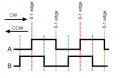With LEDs on A and B signals you should see something like this as you slowly turn the wheel.

A B
0 0
1 0
1 1
0 1
0 0
1 0
etc

or this while rotating in opposite direction

A B
0 0
0 1
1 1
1 0
0 0
0 1
etc.

Joined Jul 18, 2013
22,011
There are two resistors on the board so I suspect nothing else will be needed.
Rather than just two, I would have expected either one or three, the third one for the LED limiter.Max.

#### AlbertHall

Joined Jun 4, 2014
10,778
Rather than just two, I would have expected either one or three, the third one for the LED limiter.Max.
Ah! There are two sensors in a quad encoder, so two LEDs, so probably the two resistors are for the LEDs. So no help with whether pullups are needed.
Measuring the voltage on the outputs will reveal that.

Joined Jul 18, 2013
22,011
Ah! There are two sensors in a quad encoder, so two LEDs, so probably the two resistors are for the LEDs. So no help with whether pullups are needed.
Measuring the voltage on the outputs will reveal that.
It is a Slot Opto so usually there are two resistors as pull-ups for each of the open collector(s) and ONE for the LED light source.Although a blow-up of the encoder does show two LED emitters.
That is about the maximum resolution for a slotted disk, before having to use a Moiré window.
Max.

Last edited:

#### grgDek

Joined Mar 20, 2020
15
Hi guys !
Some news about my encoder :

With this DIY logic analyser from arduino https://create.arduino.cc/projecthub/vincenzo-g/diy-logic-analyzer-f61ee5,nothing append on the interface, i mean no signal, even if i turned it sloOoOOoowwly and @MrChips i do not really understand how to build a logic state monitor.. I tried to wire a LED+ on A and an other LED+ on B and then power up the encoder, turn slowly, but, nothing.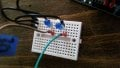I tried with the voltmeter, (-) on pin output1 and (+) on pin output2, in volt mode i always get a 1., in resistor mode (ohm), i get 131.0 Ohm, when i turn CW this value decrease, when i turn CCW it decrease too. (-) on output2 and (+) on output1 gave me 1. So i don't know how to deal with this value, is it valuable ?

I (re) tried this code :
C++:
/*     Arduino Rotary Encoder Tutorial

*
*      by Dejan Nedelkovski, www.HowToMechatronics.com
*      */

#define outputA 6
#define outputB 5
int counter = 0;
int aState;
int aLastState;
void setup() {
pinMode (outputA,INPUT_PULLUP);
pinMode (outputB,INPUT_PULLUP);

Serial.begin (9600);
// Reads the initial state of the outputA
}
void loop() {
// If the previous and the current state of the outputA are different, that means a Pulse has occured
if (aState != aLastState){
// If the outputB state is different to the outputA state, that means the encoder is rotating clockwise
counter ++;
} else {
counter --;
}
Serial.print("Position: ");
Serial.println(counter);
}
aLastState = aState; // Updates the previous state of the outputA with the current state
}
When i turn VERY slowly, ASAIC, nothing append in the serial monitor, but when i unplug the pin 6 (outputA), i have : "position : 1", when i (re)plug it : "position : 0". If i unplug or plug the outputB nothing append.

I ended up burning my encoder by trying various methods and connections to make it talk. But fortunatly my Pixma Printer has three of them. So I tried out codes and everything with a new one, (thinking that maybe the other one was damaged), but i got the same results.

I am an autodidact in electronics and programming, since 2 years, so maybe I missed something obvious ...
Could anyone explain to me how to get this encoder talk to me ? Or some tricks.

Thank you for read all this again :─)
Have a good sunday !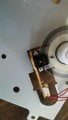Joined Jul 18, 2013
22,011
The fact that the sensor overheated indicates that maybe the LED resistors were missing.
Also generally the outputs require pull ups.
Did you reverse-engineer the circuit on the sensor board, also is there a part No. on the opto sensor itself?
Max

#### grgDek

Joined Mar 20, 2020
15
The fact that the sensor overheated indicates that maybe the LED resistors were missing.
Also generally the outputs require pull ups.
Did you reverse-engineer the circuit on the sensor board, also is there a part No. on the opto sensor itself?
Max